# NCERT Solutions for Class 10 Maths Chapter 12 Areas Related to Circles Ex 12.3

Get Free NCERT Solutions for Class 10 Maths Chapter 12 Ex 12.3 PDF. Areas Related to Circles Class 10 Maths NCERT Solutions are extremely helpful while doing your homework. Exercise 12.3 Class 10 Maths NCERT Solutions were prepared by Experienced ncert-books.in Teachers. Detailed answers of all the questions in Chapter 12 Maths Class 10 Areas Related to Circles Exercise 12.3 provided in NCERT TextBook.NCERT Solutions for Class 10 Maths Chapter 12 Areas Related to Circles Ex 12.3

NCERT Solutions for Class 10 Maths Chapter 12 Areas Related to Circles Ex 12.3 are part of NCERT Solutions for Class 10 Maths. Here we have given NCERT Solutions for Class 10 Maths Chapter 12 Areas Related to Circles Ex 12.3

[Unless stated otherwise, use$\pi =\frac { 22 }{ 7 }$.]

Question 1.
Find the area of the shaded region in the given figure, if PQ = 24 cm, PR = 7 cm and O is the centre of the circle.Solution: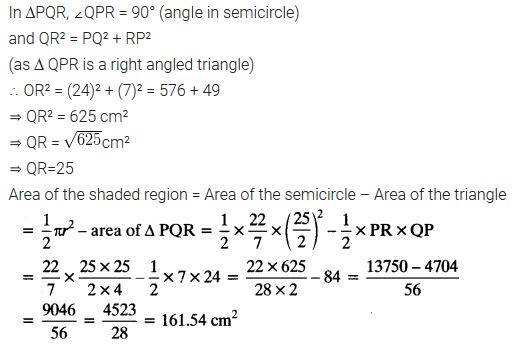Question 2.
Find the area of the shaded region in the given figure, ¡f radii of the two concentric circles with centre O are 7 cm and 14 cm respectively and ∠AOC = 40°.Solution:Question 3.
Find the area of the shaded region in the given figure, if ABCD is a square of side 14 cm and APD and BPC are semicircles.Solution:Question 4.
Find the area of the shaded region in the figure, where a circular arc of radius 6 cm has been drawn with vertex O of an equilateral triangle OAB of side 12 cm as centre.Solution: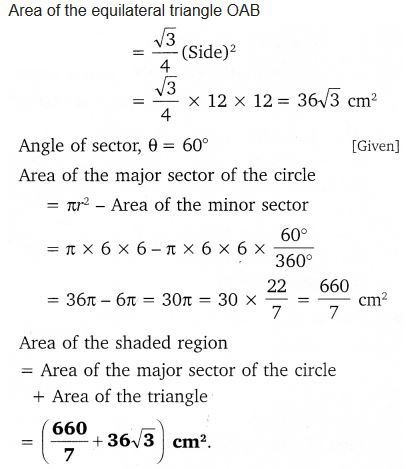Question 5.
From each corner of a square of side 4 cm a quadrant of a circle of radius 1 cm is cut and also a circle of diameter 2 cm is cut as shown in the figure. Find the area of the remaining portion of the square.Solution: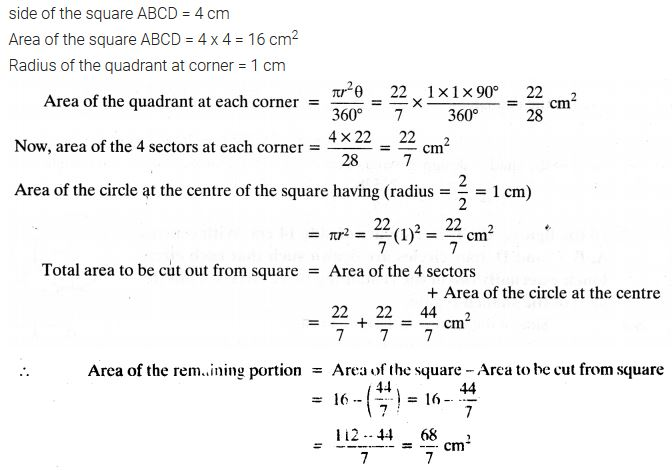Question 6.
In a circular table cover of (he radius 32 cm, a design Is formed leaving an equilìtcral triangle ABC in the middle as shown in the figure. Find the area of the design (shaded region).Solution: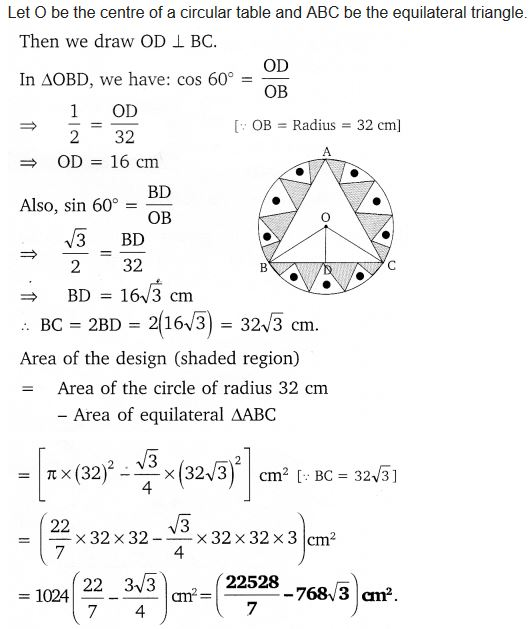Question 7.
In the figure, ABCD is a square of side 14 cm. With centres A, B, C and D, four circles are drawn such that each circle touch externally two of the remaining three circles. Find the area of the shaded region.
Solution:Question 8.
The given figure depicts a racing track whose left and right ends are semicircular. The distance between the two inner parallel line segments is 60 m and they are each 106 m long. If the track is 10 m wide, find:(i) the distance around the track along its inner edge.
(ii) the area of the track.
Solution: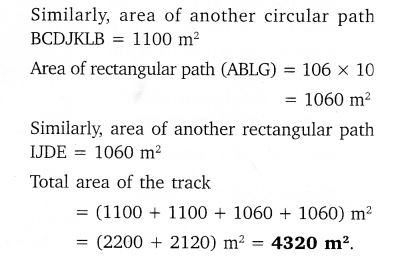Question 9.
In the figure, AB and CD are two diameters of a circle (with centre O) perpendicular to each other and OD is the diameter of the smaller circle. If OA = 7 cm, find the area of the shaded region.Solution: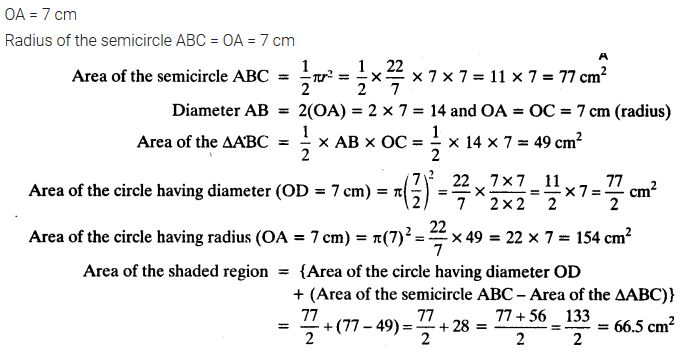Question 10.
The area of an equilateral triangle ABC is 17320.5 cm2. With each vertex of the triangle as centre, a circle is drawn with radius equal to half the length of the side of the triangle (see figure). Find the area of the shaded region.
(Use π = 3.14 and √ 3 = 1.73205).Solution:Question 11.
On a square handkerchief, nine circular designs each of the radius 7 cm are made (see figure). Find the area of the remaining portion of the handkerchief.Solution:Question 12.
In the figure, OACB is a quadrant of a circle with centre O and radius 3.5 cm. If OD = 2 cm, find the area of theSolution: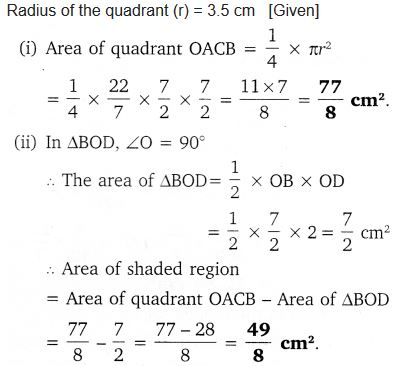Question 13.
In the figure, a square OABC is inscribed in a quadrant OPBQ. If OA = 20 cm, find the area of the shaded region. (Use π = 3.14)Solution: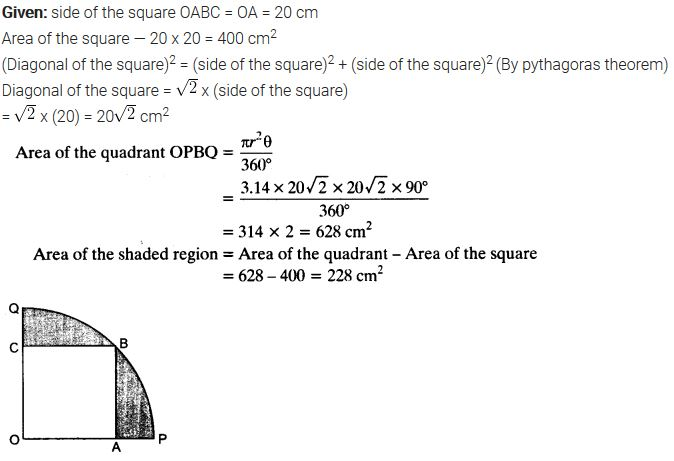Question 14.
AB and CD are respectively arcs of two concentric circles of radii 21 cm and 7 cm and centre O (see figure). If ZAOB=30°, find the area of the shaded region.Solution: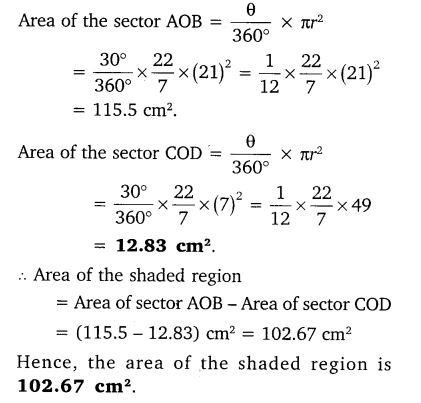Question 15.
In the figure, ABC is a quadrant of a circle of radius 14 cm and a semicircle is drawn with BC as diameter. Find the area of the shaded region.Solution: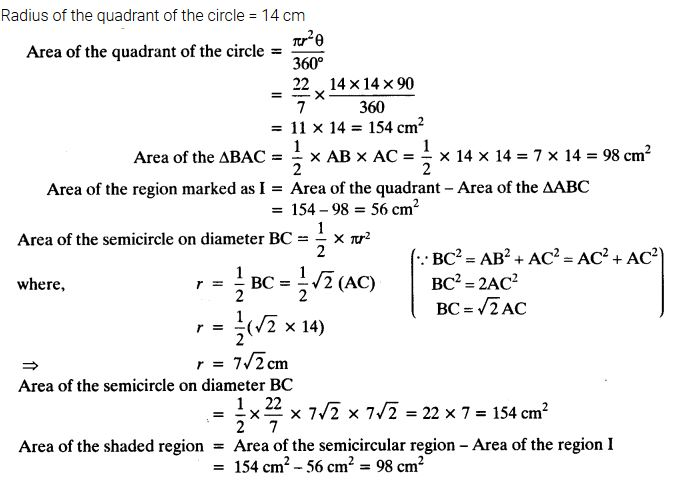Question 16.
Calculate the area of the designed region in the figure common between the two quadrants of the circles of the radius 8 cm each.Solution:+